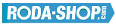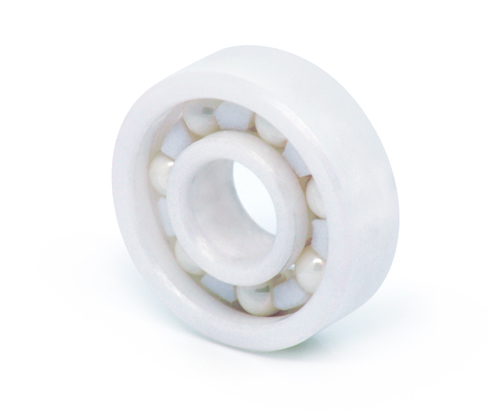# Hybrid and Ceramic BearingsManufacturer+ Product Image Item Name Price### HYB MR 115-2Z

Rodamientos Hibridos Aros Inoxidables y Bolas Cerámicas con obturaciones metálicas Dimension d=5 Dimension D=11 Dimension B=4
inside diameter = 5
outside diameter = 11
width = 4
€17.12### 6000-CE-SI3N4-PTFE

FULL CERAMIC SI3N4 BALL BEARING.PTFE CAGE
inside diameter = 10
outside diameter = 26
width = 8
€54.45### 6001-CE-SI3N4-PFTE

Solid Ceramic Bearings Ceramic Open(No Shields) Dimension d=12 Dimension D=28 Dimension B=8 Dinamic Load Rating=3060 Static Load Rating=1422 Weight=0.022gr. Speed limit Grease=N/A Speed limit Oil=0
inside diameter = 12
outside diameter = 28
width = 8### 6001-CE-ZR02-PFTE

Solid Ceramic Bearings Ceramic Open(No Shields) Dimension d=12 Dimension D=28 Dimension B=8 Dinamic Load Rating=3060 Static Load Rating=1422 Weight=0.022gr. Speed limit Grease=N/A Speed limit Oil=0 Flanged
inside diameter = 12
outside diameter = 28
width = 8
call### 6002-CE-SI3N4-PFTE

Solid Ceramic Bearings Ceramic Open(No Shields) Dimension d=15 Dimension D=32 Dimension B=9 Dinamic Load Rating=3360 Static Load Rating=1698 Weight=0.031gr. Speed limit Grease=N/A Speed limit Oil=0 Flanged
inside diameter = 15
outside diameter = 32
width = 9### 6002-CE-ZR02-PFTE

Solid Ceramic Bearings Ceramic Open(No Shields) Dimension d=15 Dimension D=32 Dimension B=9 Dinamic Load Rating=3360 Static Load Rating=1698 Weight=0.031gr. Speed limit Grease=N/A Speed limit Oil=0 Flanged
inside diameter = 15
outside diameter = 32
width = 9
call### 6003-CE-SI3N4-PFTE

Solid Ceramic Bearings Ceramic Open(No Shields) Dimension d=17 Dimension D=35 Dimension B=10 Dinamic Load Rating=3600 Static Load Rating=1950 Weight=0.041gr. Speed limit Grease=N/A Speed limit Oil=0 Flanged
inside diameter = 17
outside diameter = 35
width = 10### 6003-CE-ZR02-PFTE

Solid Ceramic Bearings Ceramic Open(No Shields) Dimension d=17 Dimension D=35 Dimension B=10 Dinamic Load Rating=3600 Static Load Rating=1950 Weight=0.041gr. Speed limit Grease=N/A Speed limit Oil=0 Flanged
inside diameter = 17
outside diameter = 35
width = 10
call### 6004-CE-SI3N4-PFTE

Solid Ceramic Bearings Ceramic Open(No Shields) Dimension d=20 Dimension D=42 Dimension B=12 Dinamic Load Rating=5640 Static Load Rating=3000 Weight=0.068gr. Speed limit Grease=N/A Speed limit Oil=0 Flanged
inside diameter = 20
outside diameter = 42
width = 12### 6004-CE-ZR02-PFTE

Solid Ceramic Bearings Ceramic Open(No Shields) Dimension d=20 Dimension D=42 Dimension B=12 Dinamic Load Rating=5640 Static Load Rating=3000 Weight=0.068gr. Speed limit Grease=N/A Speed limit Oil=0 Flanged
inside diameter = 20
outside diameter = 42
width = 12
call